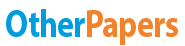# Phase III Timelines Update Predictive Modeling

Essay by   •  May 22, 2019  •  Essay  •  4,632 Words (19 Pages)  •  483 Views

## Essay Preview: Phase III Timelines Update Predictive Modeling

Report this essay
Page 1 of 19

Predictive Modeling: Exam 2 Study Guide

1. Understand each model – Use case, what can the model predict – continuous/categorical/both, input variable type – continuous/categorical/both, pros/cons, theory – how and why it works

2. Be able to calculate both Exact Naïve Bayes and Naïve Bayes prediction (multiplying fractions of probabilities).

Target variable: Audit finds fraud, no fraud

Predictors:

• Prior pending legal charges (yes/no)

• Size of firm (small/large)

3. Be able to interpret the output in JMP from screenshots.

a. Examples:

i. Linear Regression – Interpret the results and write regression equation from parameter estimates. Toyota Corolla price = left column below which corresponds to parameter estimates on right.

ii. Be able to interpret parameter estimates. Below are the parameter estimates from the linear regression of predicted the price of a Toyota Corolla.

1. Continuous variables – When interpreting the estimate column, we know that for ever 1 month increase in age the price of the car decreases by \$134.14. We also know that for ever mile the car is driven the price decreases by \$0.02 straightforward interpretation for continuous variables.

2. Categorical variables are a little more difficult to interpret because the parameter estimate shows n-1 values. Example – Metallic is 0 or 1 but only Metallic is shown below. Similarly, there are 3 fuel types, but only Fuel Type[CNG] and Fuel Type[Diesel] are listed below. Key idea: Categorical variables will always sum to zero. Therefore, a car that is not Metallic has a coefficient of 19 (from below parameter estimates) and this means a car that is Metallic has a coefficient of -19. And the price difference between a car that is metallic and is not metallic is \$38 (not \$19). For Fuel Type CNG = -933 and Diesel = -804 which means that Petrol must be positive 1737 because -933-804+1737=0

iii. Be able to interpret residuals – For the Toyota Corolla price on average the linear regression model is predicting \$110.91 too low (from Mean below) with an even distribution of errors and for the mid 50% of the model is predicting about + or - \$850.

iv. Neural Networks – Craft the formula that feeds into each node based on parameter estimates, find number of layers (one in below example), number of nodes

1. Node 3 output = Fat score 0.2*randomly assigned weight 0.05 + salt score 0.9*randomly assigned weight 0.01 + -0.3 which then goes through the transformation in the equation below and comes out as 0.43

In JMP: Node 1 input formula = fat score * 15.08 + salt score * 4.31 + -4.42

Note: The numbers won’t show on the lines in JMP, they’re just listed under Estimate

4. Variable selection methods

a. Exhaustive Search: All Possible Model (not as popular or common as forward and backward)

i. All possible subsets of predictors assessed:

1. Each individual predictor, Pairs of predictors, Sets of 3 predictors, Etc

2. Look for lowest RMSE (number 7 below)

b. Forward selection (very common method): Start with no predictor variables and add them in one by one until you achieve optimal results. You want to maximize RSquare on Validation. The best is shown at the bottom of the step history with an RSquare of 0.8774

i. Start with no predictors, add them one by one (add the one with largest contribution). P-Values change every time you include or exclude a variable.

ii. When to stop:

1. P-value Threshold: Stop when no other potential predictor has statistically significant contribution

2. Max Validation RSquare: Stop when the RSquare on the validation set stops improving when predictors are added (only available when there is a validation column)

c. Backward elimination (very common method): Start with all the predictors variables in and remove them one by one until you achieve optimal results

i. Start with all predictors, successively eliminate least useful predictors one by one. P-Values change every time you include or exclude a variable.

ii. Stopping Rules:

1. P-value Threshold: Stop when all remaining predictors have statistically significant contribution

2. Max Validation RSquare: Stop when the RSquare on the validation set stops improving when predictors are removed (only available when there is a validation column)

d. Mixed Stepwise: Remove one, add another, remove a different one.

i. Like Forward Selection, except at each step, also consider dropping non-significant predictor variables

ii. Stopping Rules

1. P-value Threshold: Stop removing when all remaining predictors are significant, and stop adding when no other potential predictor is significant

5. Understand p-values: P-Value is the probability that the Null Hypothesis is true.

a. Based around statistical testing. Base understanding is that nothing is statistically significant. Null hypothesis is that the coefficient is zero and the variable is not significant, it has no impact on the target.

i. When considering predictor variables:

1. Null Hypothesis

a. The coefficient for the variable = 0

b. The variable is not significant

2. Alternate Hypothesis

a. The coefficient is <> 0

b. The variable is significant

3. Alpha is the threshold for determining significance (cutoff)

a. Often

...

...